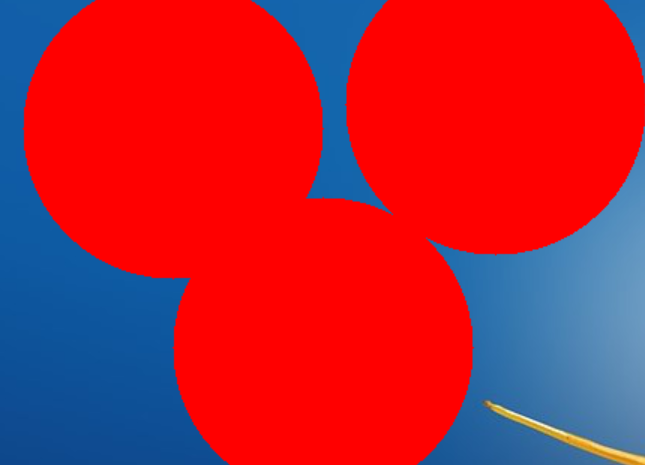# Drawing with Mouse on Images using Python-OpenCV

OpenCV is a huge open-source library for computer vision, machine learning, and image processing. OpenCV supports a wide variety of programming languages like Python, C++, Java, etc. It can process images and videos to identify objects, faces, or even the handwriting of a human. In this article, we will try to draw on images with the help of the mouse. Before learning how to draw on images using a mouse, we need to understand what is a callback.<

### Callback

A callback in programming means to call this function (the callback) when a process completes. The same applies to event-oriented programming in general. When a mouse button is clicked (an event), call a function. We don’t know when the button will be clicked. All we can do is tell the button to “call me back” or call this function when the mouse button is clicked.

### Mouse Callbacks

A callback can happen when a user performs an operation using the mouse; this operation is usually known as an event. Only one callback is present for a mouse, which is setMouseCallback(), all mouse operation will call this function only.

We can have conditional blocks to execute something based on the event/operation performed using the mouse. The mouse events/operations could be:

• EVENT_MOUSEMOVE
• EVENT_LBUTTONDOWN
• EVENT_RBUTTONDOWN
• EVENT_LBUTTONUP
• EVENT_RBUTTONUP

When should this callback occur :

We want to have this call back only when we use the mouse on the pop-up window, which has the title as “Title of Popup Window.”

```cv2.namedWindow("Title of Popup Window")
```

Example 1: Draw Circle when we left-click on a popup with OpenCV :

 `import` `cv2 ` ` `  ` `  `img ``=` `cv2.imread(``"flower.jpg"``) ` ` `  `def` `draw_circle(event, x, y, flags, param): ` `     `  `    ``if` `event ``=``=` `cv2.EVENT_LBUTTONDOWN: ` `        ``print``(``"hello"``) ` `        ``cv2.circle(img, (x, y), ``100``, (``0``, ``255``, ``0``), ``-``1``) ` `         `  `cv2.namedWindow(winname ``=` `"Title of Popup Window"``) ` `cv2.setMouseCallback(``"Title of Popup Window"``, draw_circle) ` ` `  `while` `True``: ` `    ``cv2.imshow(``"Title of Popup Window"``, img) ` `     `  `    ``if` `cv2.waitKey(``10``) & ``0xFF` `=``=` `27``: ` `        ``break` `         `  `cv2.destroyAllWindows() `

Output:Example 2: Drawing a Rectangle by dragging on Images with OpenCV

 `import` `cv2 ` ` `  ` `  `img ``=` `cv2.imread(``"flower.jpg"``) ` ` `  `# variables ` `ix ``=` `-``1` `iy ``=` `-``1` `drawing ``=` `False` ` `  `def` `draw_rectangle_with_drag(event, x, y, flags, param): ` `     `  `    ``global` `ix, iy, drawing, img ` `     `  `    ``if` `event ``=``=` `cv2.EVENT_LBUTTONDOWN: ` `        ``drawing ``=` `True` `        ``ix ``=` `x ` `        ``iy ``=` `y             ` `             `  `    ``elif` `event ``=``=` `cv2.EVENT_MOUSEMOVE: ` `        ``if` `drawing ``=``=` `True``: ` `            ``cv2.rectangle(img, pt1 ``=``(ix, iy), ` `                          ``pt2 ``=``(x, y), ` `                          ``color ``=``(``0``, ``255``, ``255``), ` `                          ``thickness ``=``-``1``) ` `     `  `    ``elif` `event ``=``=` `cv2.EVENT_LBUTTONUP: ` `        ``drawing ``=` `False` `        ``cv2.rectangle(img, pt1 ``=``(ix, iy), ` `                      ``pt2 ``=``(x, y), ` `                      ``color ``=``(``0``, ``255``, ``255``), ` `                      ``thickness ``=``-``1``) ` `         `  `cv2.namedWindow(winname ``=` `"Title of Popup Window"``) ` `cv2.setMouseCallback(``"Title of Popup Window"``,  ` `                     ``draw_rectangle_with_drag) ` ` `  `while` `True``: ` `    ``cv2.imshow(``"Title of Popup Window"``, img) ` `     `  `    ``if` `cv2.waitKey(``10``) ``=``=` `27``: ` `        ``break` ` `  `cv2.destroyAllWindows() `

Output:### What does (cv2.waitKey(10) & 0xFF == 27) do ?

cv2.waitKey() returns a 32 Bit integer value (might be dependent on the platform). The key input is in ASCII which is an 8 Bit integer value. So you only care about these 8 bits and want all other bits to be 0. This you can achieve with: `cv2.waitKey(10) & 0xFF == 27`

Whether you're preparing for your first job interview or aiming to upskill in this ever-evolving tech landscape, GeeksforGeeks Courses are your key to success. We provide top-quality content at affordable prices, all geared towards accelerating your growth in a time-bound manner. Join the millions we've already empowered, and we're here to do the same for you. Don't miss out - check it out now!

Previous
Next